Contents

# Contents

## Idea

bound state of Dp- with D(p+2)-branes.

## Properties

### Transveral Dp-D(p+2)-brane intersections in fuzzy funnels

The boundary condition in the nonabelian DBI model of coincident Dp-branes describing their transversal intersection/ending with/on D(p+2)-branes is controled by Nahm's equation and thus exhibits the brane intersection-locus equivalently as:

1. a fuzzy funnel noncommutative geometry interpolating between the $\mathrm{D}p$- and the $\mathrm{D}(p+2)$-brane worldvolumes;

2. geometric engineering of Yang-Mills monopoles in the worldvolume-theory of the ambient $D(p+2)$-branes.

More explicitly, for $y \in (0,\infty ]$ the transversal distance along the stack of $N$ $\mathrm{D}p$-branes away from the $\mathrm{D}(p+2)$-brane, and for

$X^i \in C^\infty\big( (0,\infty], \mathfrak{u}(N) \big) \phantom{AAA} i \in \{1,2,3\}$

the three scalar fields on the worldvolume, the boundary condition is:

$\frac{d}{d y} X^3 + [X^1, X^2] \;=\; 0 \,, \;\;\; \frac{d}{d y} X^1 + [X^2, X^3] \;=\; 0 \,, \;\;\; \frac{d}{d y} X^2 + [X^3, X^1] \;=\; 0$

as $y \to 0$. These are Nahm's equations, solved by

$X^i(y) = \frac{1}{y} \rho^i + \text{non-singular}$

where

$\rho \;\colon\; \mathfrak{su}(2) \longrightarrow \mathfrak{u}(N)$

is a Lie algebra homomorphism from su(2) to the unitary Lie algebra, and

$\rho^i \coloneqq \rho(\sigma^i)$

is its complex-linear combination of values on the canonical Pauli matrix basis.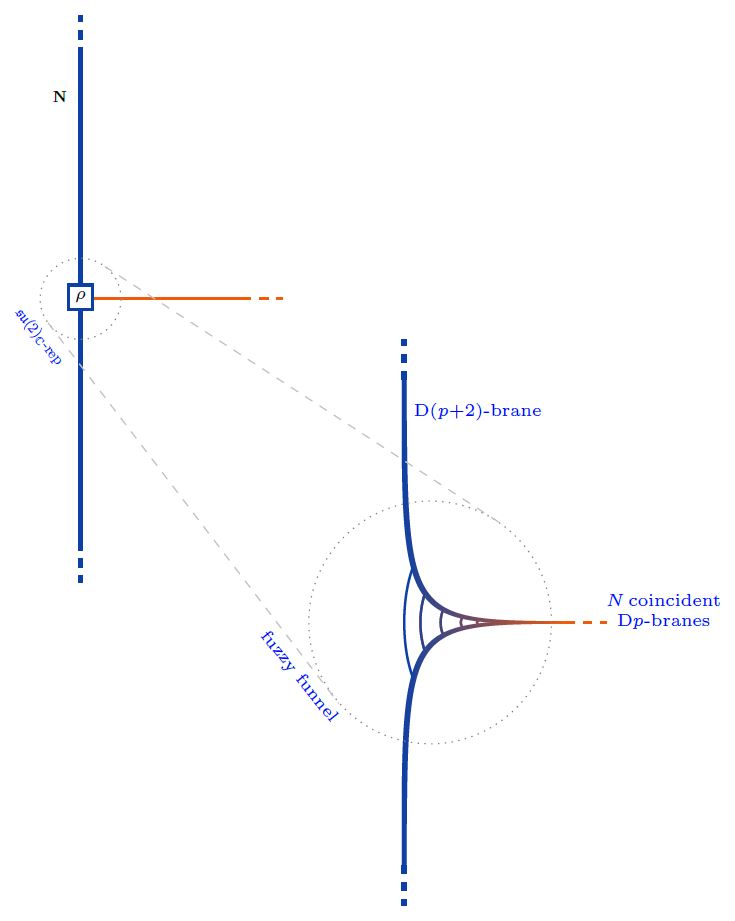Equivalently. $\rho$ is an $N$-dimensional complex Lie algebra representation of su(2). Any such is reducible as a direct sum of irreducible representations $\mathbf{N}^{(M5)}$, for which there is exactly one, up to isomorphism, in each dimension $N^{(M5)} \in \mathbb{N}$:

(1)$\rho \;\simeq\; \underset{ i }{\bigoplus} \big( N_i^{(M2)} \cdot \mathbf{N}_i^{(M5)} \big) \,.$

(Here the notation follows the discussion at M2/M5-brane bound states in the BMN model, which is the M-theory lift of the present situation).

Now each irrep $\mathbf{N}_i^{(M5)}$ may be interpreted as a fuzzy 2-sphere of radius $\propto \sqrt{ \left( N_i^{(M5)}\right)^2 - 1 }$, hence as the section of a fuzzy funnel at given $y = \epsilon$, whence the totality of (1) represents a system of concentric fuzzy 2-spheres/fuzzy funnels.

graphics from Sati-Schreiber 19c

Moreover, since the complexification of su(2) is the complex special linear Lie algebra $\mathfrak{sl}(2,\mathbb{C})$ (here) the solutions to the boundary conditions are also identified with finite-dimensional $\mathfrak{sl}(2,\mathbb{C})$ Lie algebra representations:

(2)$\rho \;\in\; \mathfrak{sl}(2,\mathbb{C}) Rep \,.$

This is what many authors state, but it is not yet the full picture:

Also the worldvolume Chan-Paton gauge field component $A$ along $y$ participates in the brane intersection

$A \in C^\infty\big( (0,\infty], \mathfrak{u}(N) \big)$

its boundary condition being that

$[A, X^i] \;=\; 0 \phantom{AAAA} \text{for all}\; i \in \{1,2,3\}$

as $y \to 0$ (Constable-Myers 99, Section 3.3, Thomas-Ward 06, p. 16, Gaiotto-Witten 08, Section 3.1.1)

Together with (2) this means that the quadruple of fields $(X^1,X^2,X^3,A)$ constitutes a Lie algebra representation of the general linear Lie algebra

$\mathfrak{gl}(2,\mathbb{C}) \;\simeq\; \underset{ \langle X^1, X^2, X^3 \rangle }{ \underbrace{ \mathfrak{sl}(2,\mathbb{C}) } } \oplus \underset{ \langle A \rangle }{ \underbrace{ \mathbb{C} } }$

This makes little difference as far as bare Lie algebra representations are concerned, but it does make a crucial difference when these are regarded as metric Lie representations of metric Lie algebras, since $\mathfrak{gl}(2,\mathbb{C})$ admits further invariant metrics…

### $\mathrm{D}6 \perp \mathrm{D}8$-brane intersections

Specifically for $p = 6$, i.e. for D6-D8 brane intersections, this fits with the Witten-Sakai-Sugimoto model geometrically engineering quantum chromodynamics, and then gives a geometric engineering of the Yang-Mills monopoles in actual QCD (HLPY 08, p. 16).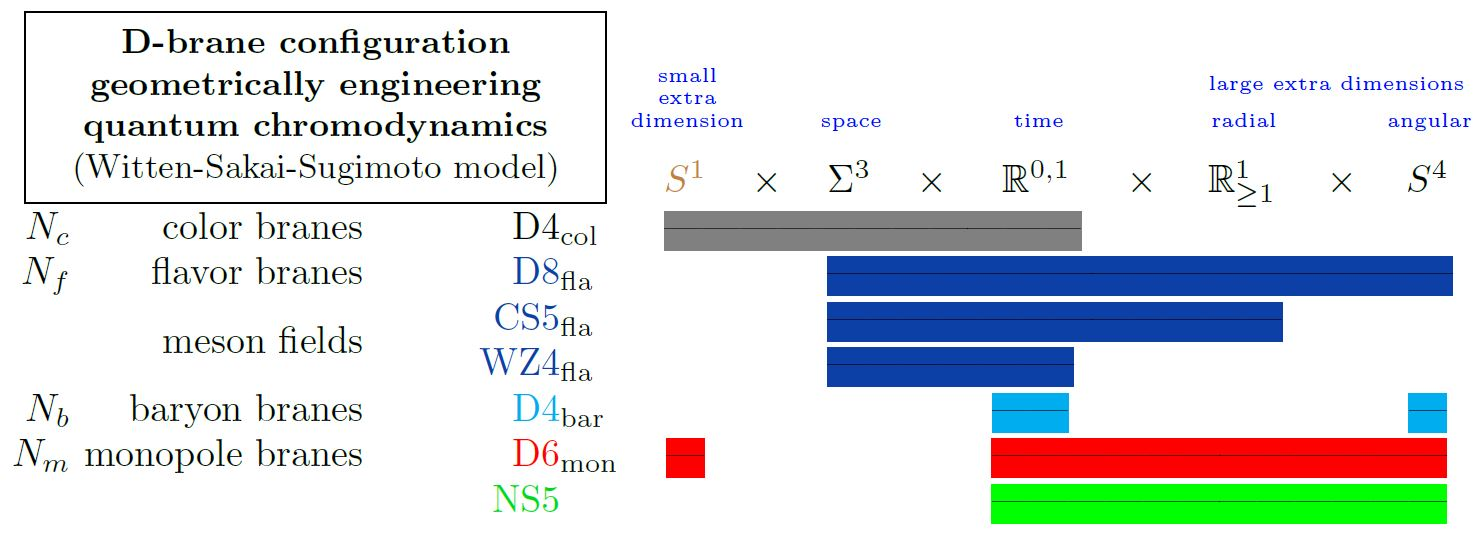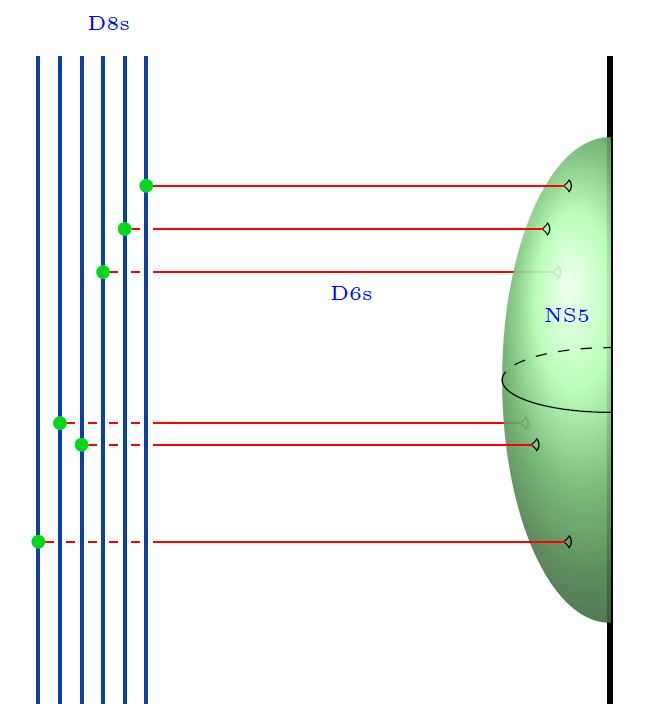Here we are showing

1. the color D4-branes;

2. the flavor D8-branes;

with

1. the 5d Chern-Simons theory on their worldvolume

2. the corresponding 4d WZW model on the boundary

both exhibiting the meson fields

3. (see below at WSS – Baryons)

4. (see at D6-D8-brane bound state)

5. the NS5-branes.

### The s-rule

What has come to be known as the s-rule is the conjecture that the configuration of Dp-D(p+2)-brane bound states with the Dp-branes stretching from the D(p+2)-branes to NS5-branes, can be supersymmetric only if at most one D$p$-brane ends on any one D$(p+2)$-brane.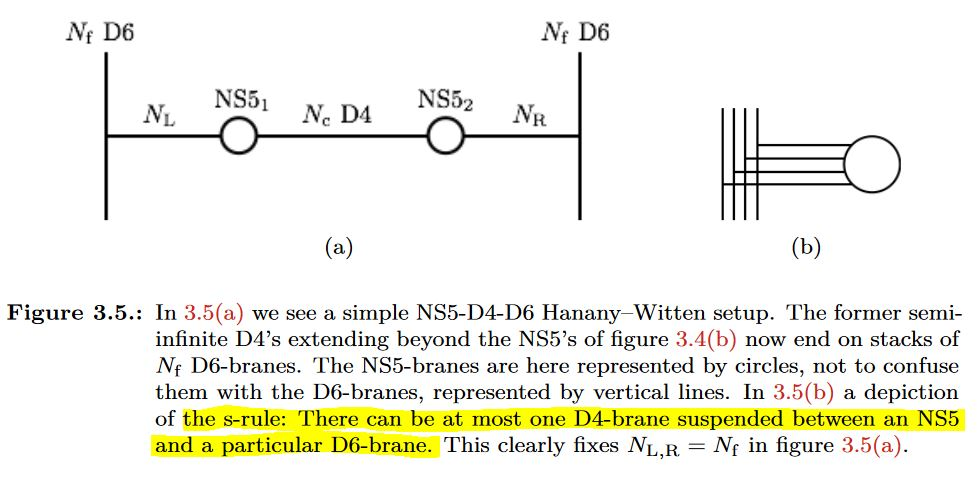graphics grabbed from Fazzi 17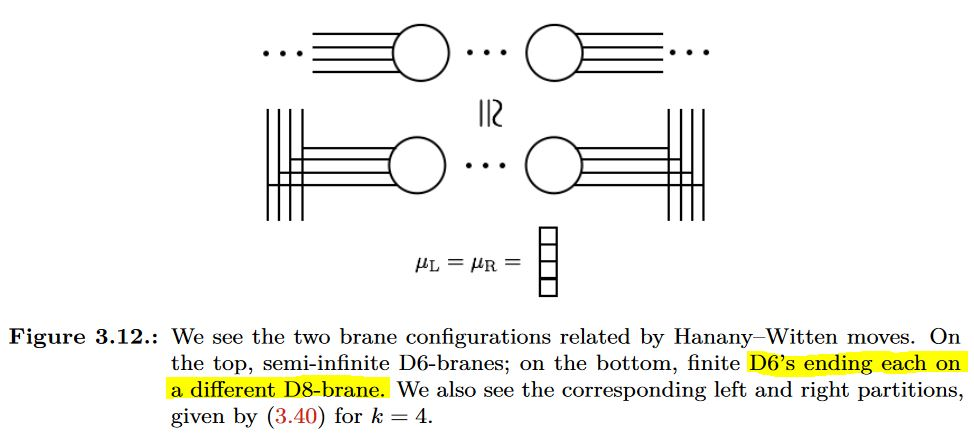graphics grabbed from Fazzi 17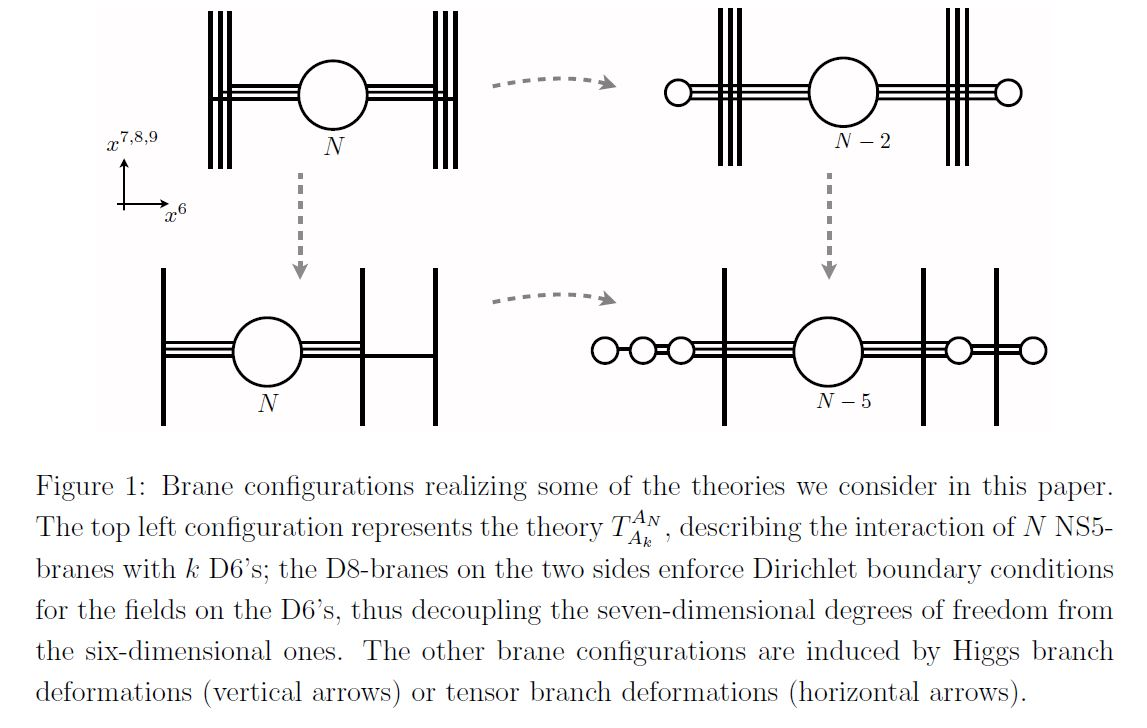graphics grabbed from Gaiotto-Tomasiello 14

### Single trace observables as $\mathfrak{su}(2)$-weight systems on chord diagrams

We discuss how the single trace observables on the fuzzy 2-sphere-sections of Dp-D(p+2) brane intersection fuzzy funnels are given by su(2)-Lie algebra weight systems on chord diagrams (following Ramgoolam-Spence-Thomas 04, McNamara-Papageorgakis 05, see McNamara 06, Section 4 for review).

For more see at weight systems on chord diagrams in physics.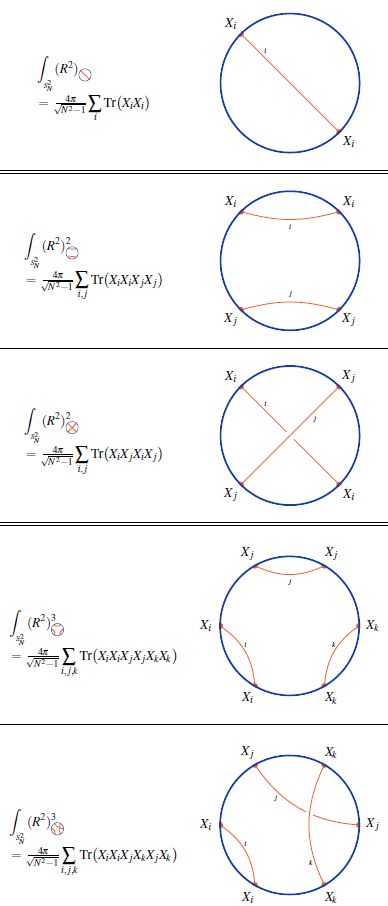graphics from Sati-Schreiber 19c

While in the commutative large N limit, all powers of the radius function on the fuzzy 2-sphere are equal

$\underset{N\to \infty}{\lim} \int_{S^2_N} R^{2 k} \;=\; 4 \pi \,;$

for finite $N$ there is an ordering ambiguity: In fact, the number of functions on the fuzzy 2-sphere at finite $N$ that all go to the same function $R^{2k}$ in the large N limit grows rapidly with $k$.

At $k = 1$ there is the single radius observable (?)

$\int_{S^2_N} R^2 \;=\; \int_{S^2_N} \underset{i}{\sum} X_i \cdot X_i \;=\; 4 \pi \tfrac{ N }{ \sqrt{N^2 -1} }$

At $k = 2$ there are, under the integral (?), two radius observables:

1. $\int_{S^2_N} \underset{i,j}{\sum} X_i X_i X_j X_j$

2. $\int_{S^2_N} \underset{i,j}{\sum} X_i X_j X_j X_i$

(Here we are using that under the integral/trace, a cyclic permutation of the factors in the integrand does not change the result).

Similarly for higher $k$, where the number of possible orderings increases rapidly. The combinatorics that appears here is familiar in knot theory:

Every ordering of operators, up to cyclic permutation, in the single trace observable $Tr(R^2)^n$ is encoded in a chord diagram and the value of the corresponding single trace observable is the value of the su(2)-Lie algebra weight system on this chord diagram.

### Parallel intersection

brane intersections/bound states/wrapped branes/polarized branes

S-duality$\,$bound states:

intersecting$\,$M-branes:

### For parallel intersection

For parallel intersection:

• E. Gava, K.S. Narain, M.H. Sarmadi, On the Bound States of $p$- and $(p+2)$-Branes, Nucl. Phys. B504 (1997) 214-238 (arxiv:hep-th/9704006)

### For transversal intersections

#### As spikes/BIons

On Dp-D(p+2) brane intersections as spikes/BIons

from the M5-brane:

#### As fuzzy funnels/Yang-Mills monopoles

On transversal Dp-D(p+2) brane intersections as Yang-Mills monopoles / fuzzy funnel-solutions to Nahm's equation:

For transversal D1-D3 brane intersections:

For transversal D2-D4-brane bound states (with an eye towards AdS/QCD):

• Alexander Gorsky, Valentin Zakharov, Ariel Zhitnitsky, On Classification of QCD defects via holography, Phys. Rev. D79:106003, 2009 (arxiv:0902.1842)

For transversal D3-D5 brane intersections:

For transversal D6-D8 brane intersections (with an eye towards AdS/QCD):

• Deog Ki Hong, Ki-Myeong Lee, Cheonsoo Park, Ho-Ung Yee, Section V of: Holographic Monopole Catalysis of Baryon Decay, JHEP 0808:018, 2008 (https:arXiv:0804.1326)

and as transversal D6-D8-brane bound states on a half NS5-brane in type I' string theory:

Making explicit the completion of the $\mathfrak{su}(2)_{\mathbb{C}} \simeq \mathfrak{sl}(2,\mathbb{C})$-representation to a $\mathfrak{gl}(2,\mathbb{C})$-representation by adjoining the gauge field component $A_y$ to the scalar fields $\vec X$:

#### Relation to Vassiliev braid invariants

Relation of Dp-D(p+2)-brane bound states (hence Yang-Mills monopoles) to Vassiliev braid invariants via chord diagrams computing radii of fuzzy spheres:

#### Lift to M2-M5-brane bound states

The lift of Dp-D(p+2)-brane bound states in string theory to M2-M5-brane bound states/E-strings in M-theory, under duality between M-theory and type IIA string theory+T-duality, via generalization of Nahm's equation (this eventually motivated the BLG-model/ABJM model):

### Single trace observables as weight systems on chord duagrams

Relation of single trace observables in the non-abelian DBI action on Dp-D(p+2)-brane bound states (hence Yang-Mills monopoles) to su(2)-Lie algebra weight systems on chord diagrams computing radii averages of fuzzy spheres: